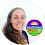## Pages

### Free Exponential Functions Flowchart

One of the hardest things about learning the different exponential functions is knowing when to use each one. I shared a graphing exponential functions cheat sheet the other day. The flowchart in this post is to help students identify which exponential function a word problem is asking them to use. Some of our Algebra 2 students didn't need it, others found it super helpful.

The flowchart starts by asking students if their word problem mentions the word compounded. This is a good way to immediately differentiate between exponential word problems involving bank interest and the other types of exponential word problems.
From there, one side of the flowchart leads students to the number of times interest is compounded per year while the other side of the flowchart moves into the half-life, appreciation and depreciation equations.

Later in the year we graph rational functions. During this unit, I find students need extra help with the steps. If you have found that graphing rationals is difficult for your students, you may like this post with a reference sheet for graphing rationals.

You can find the exponential functions flowchart free hereMORE FREE MATH CHEAT SHEETS

1.1.